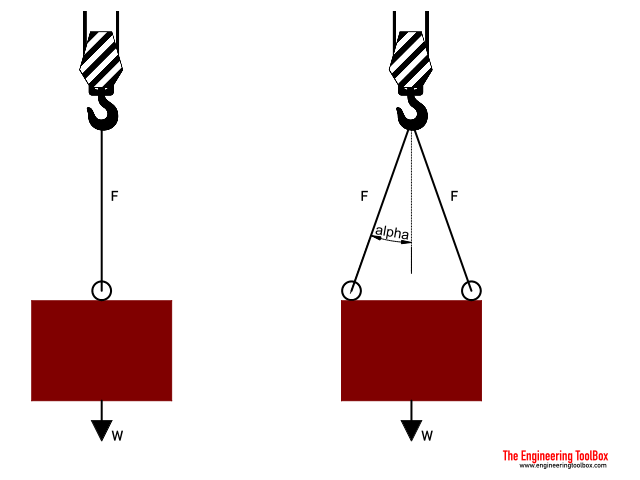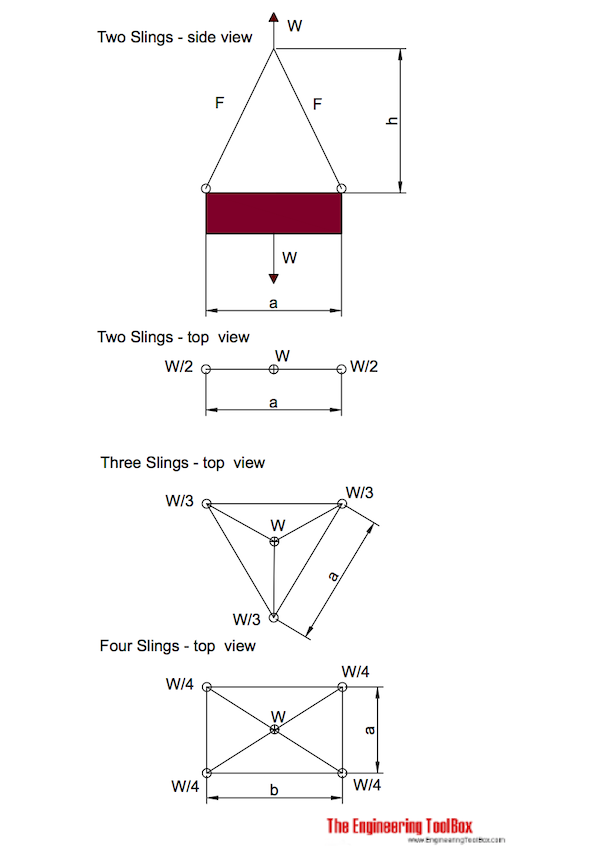Engineering ToolBox - Resources, Tools and Basic Information for Engineering and Design of Technical Applications!

# Wire Rope Slings

## Sling angles and influence on capacity.

Slings angles affects ropes capacities.If angle - alpha - is measured between

• the vertical line (as with gravity force), and
• the rope or wire

the relative capacity compared to a vertical straight lifting is reduced with reduction factor as indicated below.

f = cos(α)                           (1)

where

f = reduction factor

α = angle between vertical line and rope (degrees)

Angle
- α -
(degrees)
Reduction Factor
- f -
0 1.000
10 0.985
20 0.940
30 0.866
40 0.766
50 0.643
60 0.500
70 0.342

### Example - Capacity of a Single Rope or Wire

The capacity of a single rope that follows a vertical line is 100% since the reduction factor is 1.

If the weight of a body is W - the load in the wire is

F = W                                     (2)

where

F = force in rope (N, lbf)

W = m g = weight of body (N, lbf)

m = mass of body (kg, slugs)

g = acceleration of gravity (9.81 m/s2, 32.17 ft/s2)

For a body with mass 100 kg the load in the rope can be calculated

F = (100 kg) (9.81 m/s2)

= 981 N

= 9.8 kN

### Example - Capacity of Two Ropes (or Wires)

#### Two wires or ropes follows the vertical line

The capacity of two wires that follows the vertical line is 100% since the reduction factor is 1.

If the weight of a body is W - the load in each wire is

F = W / 2                                  (3)

For a body with weight 1000 N the load in each rope can be calculated as

F = (1000 N) / 2

= 500 N

= 0.5 kN

#### Two wires - or ropes - with angle 30o to the vertical line

The capacity of two wires with angle 30o to the vertical line is 86.6% since the reduction factor is 0.866.

If the weight of a body is W - the load in each wire is

F = (W / 2) / cos(30o)

= (W / 2) / f

= (W / 2) / 0.866

= 0.577 W                                          (4)

For a body with weight 1000 N the loads in the ropes can be calculated

F = 0.577 (1000 N)

= 577 N

= 0.58 kN

### Wire Rope Slings Calculators

The calculators below can be used to calculate wire rope forces. Note that mass (kg) and not weight (N) is used as input.mass (kg)

length - a (m)

height - h (m)

mass (kg)

length - a (m)

height - h (m)

mass (kg)

length - a (m)

length - b (m)

height - h (m)

## Related Topics

• ### Mechanics

Forces, acceleration, displacement, vectors, motion, momentum, energy of objects and more.
• ### Statics

Loads - forces and torque, beams and columns.

## Related Documents

• ### Bollard Forces

Friction, load and effort forces acting in ropes turned around bollards.

Force and tension in cables with uniform loads.
• ### Factors of Safety - FOS

Factors of Safety - FOS - are important in engineering designs.
• ### Forces and Tensions in Ropes due to Angle

Reduced load capacities in ropes, cables or lines - due to acting angle.
• ### Law of Cosines

One side of a triangle when the opposite angle and two sides are known.
• ### Manila Ropes - Strength

Minimum breaking strength and safe load of manila 3-strand rope.
• ### Nylon Ropes - Strengths

Nylon 3-strand and 8-strand rope - minimum breaking strength and safe load.
• ### Polyester Polyolefin Ropes - Strengths

Polyester Polyolefin dual fiber 3-strand rope - minimum breaking strength and safe load.
• ### Polyester Ropes - Strengths

Polyester 3-strand and 8-strand rope - minimum breaking strength and safe load.
• ### Polypropylene Fiber Ropes - Strengths

Polypropylene fiber 3-strand and 8 strand rope - minimum breaking strength and safe load.
• ### Pulleys

Pulleys, blocks and tackles.

• ### Sisal Ropes - Strengths

Sisal rope 3-strand, minimum breaking strength and safe load.
• ### Winches

Effort force to raise a load.
• ### Wire Ropes - Strengths

6 strand x 19 wire (6x19) - minimum breaking strength, safe loads and weight.

## Engineering ToolBox - SketchUp Extension - Online 3D modeling!

Add standard and customized parametric components - like flange beams, lumbers, piping, stairs and more - to your Sketchup model with the Engineering ToolBox - SketchUp Extension - enabled for use with older versions of the amazing SketchUp Make and the newer "up to date" SketchUp Pro . Add the Engineering ToolBox extension to your SketchUp Make/Pro from the Extension Warehouse !

We don't collect information from our users. More about

## Citation

• The Engineering ToolBox (2010). Wire Rope Slings . [online] Available at: https://www.engineeringtoolbox.com/wire-rope-slings-d_1613.html [Accessed Day Month Year].

Modify the access date according your visit.

9.19.12